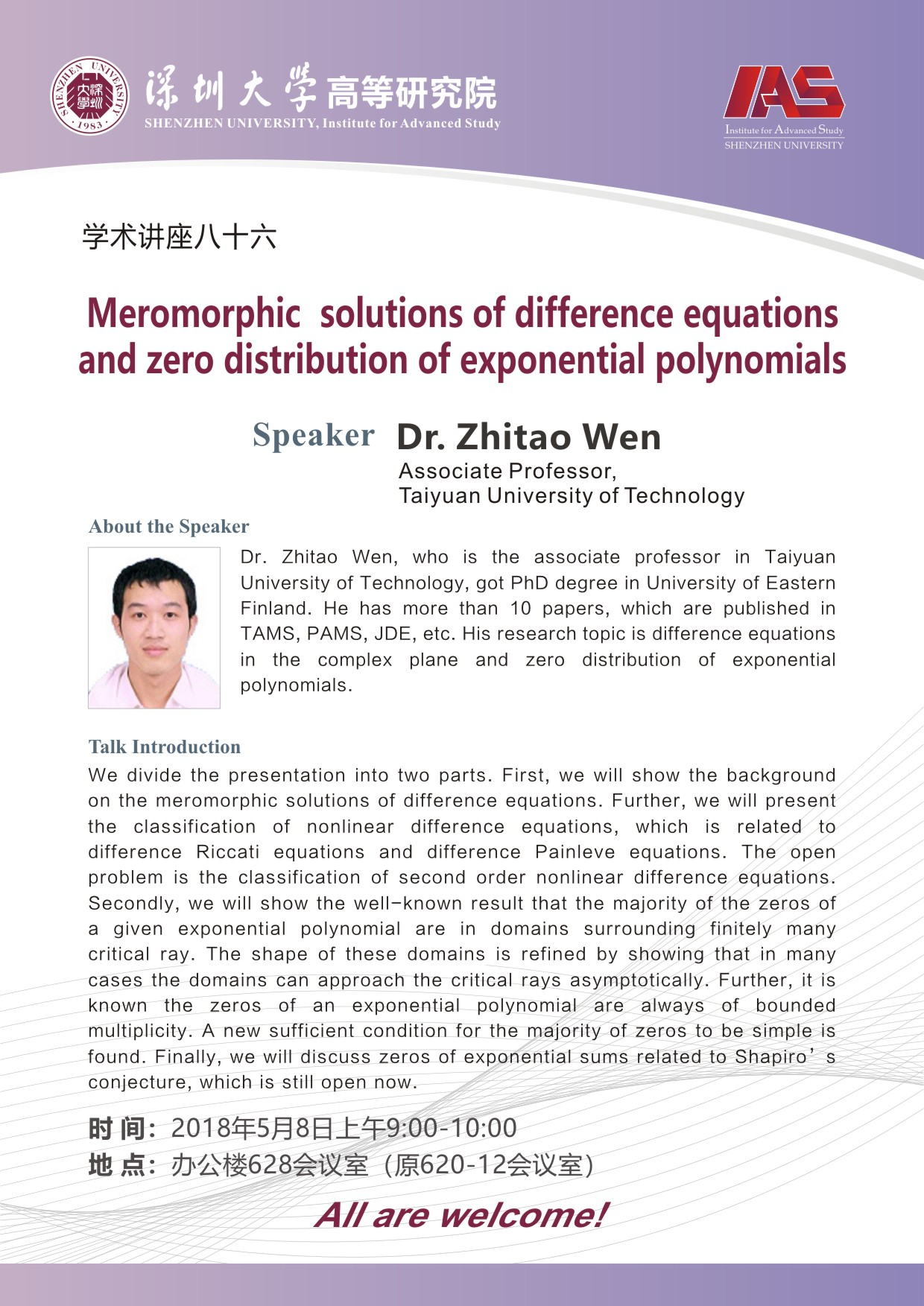﻿ 高等研究院系列学术讲座之八十六-深圳大学高等研究院

# 高等研究院系列学术讲座之八十六

Meromorphic solutions of difference equations and zero distribution of exponential polynomials

Dr. Zhitao Wen

Associate Professor, Taiyuan University of Technology

Dr. Zhitao Wen, who is the associate professor in Taiyuan University of Technology, got PhD degree in University of Eastern Finland. He has more than 10 papers, which are published in TAMS, PAMS, JDE, etc. His research topic is difference equations in the complex plane and zero distribution of exponential polynomials.

Talk Information

We divide the presentation into two parts. First, we will show the background on the meromorphic solutions of difference equations. Further, we will present the classification of nonlinear difference equations, which is related to difference Riccati equations and difference Painleve equations. The open problem is the classification of second order nonlinear difference equations. Secondly, we will show the well-known result that the majority of the zeros of a given exponential polynomial are in domains surrounding finitely many critical ray. The shape of these domains is refined by showing that in many cases the domains can approach the critical rays asymptotically. Further, it is known the zeros of an exponential polynomial are always of bounded multiplicity. A new sufficient condition for the majority of zeros to be simple is found. Finally, we will discuss zeros of exponential sums related to Shapiro’s conjecture, which is still open now.

All are welcome！